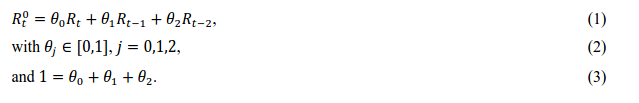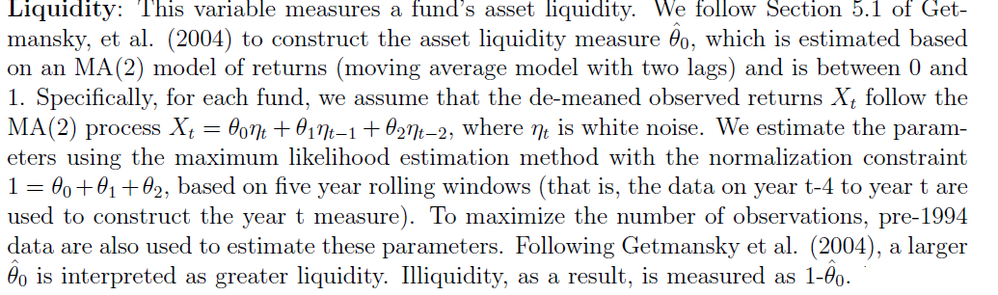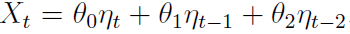## MA(2)

Re: MA(2)[ Edited ]

Hi

I am applying a second order moving average process (MA(2)) to uncover the
unobserved returns.I am trying to estimate the parameters of the model for each hedge fund strategy by maximum likeli-
hood. Then the estimated parameters will be used to desmooth returns.

My codes so far just gives me the moving average factor

proc arima data = sample ;
by mainstrategy;

identify var=returns nlag=6 outcov=acf noprint ;

estimate q=2;

run ;

Is there a problem with using the by statement in arima procedure. Is there another way to achieve the same results without using the by statement.

My orginal data is too huge to upload so here is a sample

Date       Return     AUM    mainstrategy

199512   -0.0055   26.9     Relative value

199601    0.0048   27.1      Relative value

199602    0.0089   30.7      CTA3 REPLIES 3

## Re: MA(2)

Hello,

There is no problem with using BY groups in PROC ARIMA.  In order to obtain ML estimates of the MA parameters you must use method=ml option in the estimate statement.  However, I do not understand your question very well.  It is unclear what you mean by "desmoothing" using the estimated MA parameters.  Assuming "returns" are your observed returns, you are fitting a returns = constant + MA(2) model.  If you want, (returns - estimated constant) can surve as an estimate of the MA part.  Is this what you want?

By the way, your mathematical equation does not conform to your ARIMA model specification.  Do you mean the unobserved R_t acts like the white noise part of the ARIMA model?  ARIMA assumes theta_0 to be 1.

There is another procedure, PROC UCM, in SAS/ETS that might be more appropriate for decomposing your returns into a slow moving smooth part and a rougher noise part.  See an example of trend removal using HP filter: https://support.sas.com/documentation/cdl/en/etsug/68148/HTML/default/viewer.htm#etsug_ucm_examples0...  You can also get a decomposition based on an MA(2) component (see the syntax for IRREGULAR component: https://support.sas.com/documentation/cdl/en/etsug/68148/HTML/default/viewer.htm#etsug_ucm_syntax11....).  UCM is a very general purpose procedure to obtain such decompositions.  It supports BY processing also.  If you want even more customization, you can use the SSM procedure (https://support.sas.com/documentation/cdl/en/etsug/68148/HTML/default/viewer.htm#etsug_ssm_gettingst...).  However, SSM procedure usually requires more coding.

Hope this helps.

Hope this helps.

Rajesh

## Re: MA(2)

Hi Rajesh,

I think there can be a bit of confusion in the equation because of the R's. But lets take a clearer one wherewhere X_t is the unobserved returns and  nt is the white noise. then I want to estimate the parameters using the
maximum likelihood estimation method with the normalization constraint 1 = theta0 + theta1 + theta2.

thanks

## Re: MA(2)

Unfortunately, PROC ARIMA will not be able to impose this restriction on thetas.  I think your theta_0 can be absorbed in the variance parameter of eta.  In ARIMA, theta1 and theta2 (scaled by theta0) satisfy the invertibility condition.

Discussion stats
• 3 replies
• 1093 views
• 1 like
• 2 in conversation